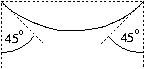Mathematical and Physical Journal
for High Schools
Issued by the MATFUND Foundation
 Already signed up? New to KöMaL?

# Exercises and problems in PhysicsOctober 2001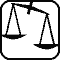## Experimental problem

M. 227. A ring hanging on a nail can swing in several ways. Take among these two characteristic ones: the one when the ring swings in its own plane, and the one perpendicular to it. Measure the two swing periods, and calculate the ratio of the periods. Give an estimation of the error of this result. (6 points)## Theoretical problems

P. 3456.  There is a motorway a railway track and a cycling path running parallel with each other. The railway track is in a 600 m, and the cycling path is in a 800 m distance of the middle positioned motorway. The velocity of the train is 90 km/h and the velocity of the car is 60 km/h. All the three vehicles are going in the same direction at a constant speed,in a way that at a given moment they fit on one line. What is the velocity of the bicycle if this situation maintains? (4 points)

P. 3457.  A vessel is completely full of a liquid of a temperature of 0 Co and of greater density than that of water. There is a piece of ice of a temperature of 0 Co floating in it. The temperature is kept constant and the piece of ice is melted by an infrared laser beam. Does the water spill over the brim? (3 points)

P. 3458.  What is the correct explanation of the phenomenon that if we put the sharp edge of an axe on a wooden clog even with an additional weight put on top of it, the surface of the clog is hardly scratched, but if we lift the axe and strike down, the clog cleaves asunder? (3 points)

P. 3459.  There are two blocks with masses of m1=0,6 kg and m2=0,4 kg lying on a horizontal surface, connected loosely by a string. We begin to pull the block of mass m1 with a F=3 N force and observe the acceleration of the bodies. We execute the experiment on surfaces of different friction coefficient. The static and kinetic friction coefficients are considered the same and $\displaystyle mu$ can vary from zero to 0,9.

a) Calculate and plot the acceleration of the bodies as a function of $\displaystyle mu$.

b) Determine and plot the dependence of the force acting in the string on the friction coefficient. (5 points)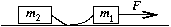P. 3460.  To determine the velocity of a bullet we put a wooden cube of mass M=1,2 kg on the edge of a table of a height of h=0,8 m and we shoot a bullet of mass m=20 g into it from a horizontal direction. (The bullet remains in the wooden cube.) As the effect of the collision the cube is thrown off the table and reaches the ground at x=3 m distance. What was the velocity of the bullet? (4 points)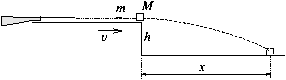P. 3461.  In the arrangement seen in the figure C can be replaced by an arbitrary capacitor. In what interval can the capacity change between points A and B due to changes in C? (5 points)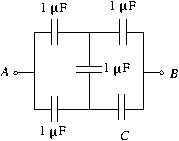P. 3462.  Batteries of the same electromotive force and internal resistance were connected first in parallel then in series with a consumer of the resistance of 129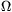. In the serial arrangement the output voltage of one battery was found 10 % less than in the parallel arrangement.

a) How many batteries were used?

b) In which case was the power of the consumer greater? (4 points)

P. 3463.  A monatomic ideal gas is compressed to one seventh of its volume while its pressure increases to seven times its original value in a process of which the image on the p-V diagram is a line segment. In which part does the gas absorb and in which part does it dissipate heat. What is the ratio of the lengths of the corresponding two intervals on the p-V diagram? (5 points)

P. 3464.  If a gas is heated, sooner or later it will emit light. Why? (4 points)

P. 3465.  A long narrow cylindrical spring is so week that it stretches considerably even under its own weight. In one case the spring is held by one of its ends and hung vertically, in another both ends are held in a distance so that the spring takes the shape shown in the figure. In which case is the spring stretched longer? (6 points)